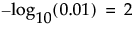Shows the LogWorth for each model effect, defined as -log10(p-value). This transformation adjusts p-values to provide an appropriate scale for graphing. A value that exceeds 2 is significant at the 0.01 level (because).
Shows the False Discovery Rate LogWorth for each model effect, defined as -log10(FDR PValue). This is the best statistic for plotting and assessing significance. Select the FDR check box to replace the LogWorth column with the FDR LogWorth column.
Shows the p-value for each model effect. This is the p-value corresponding to the significance test displayed in the Likelihood Ratio Tests report.
Shows the False Discovery Rate p-value for each model effect calculated using the Benjamini-Hochberg technique. This technique adjusts the p-values to control the false discovery rate for multiple tests. Select the FDR check box to replace the PValue column with the FDR PValue column.

Help created on 9/19/2017